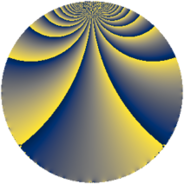# Properties

 Label 84.2.nLevel $84$ Weight $2$ Character orbit 84.n Rep. character $\chi_{84}(11,\cdot)$ Character field $\Q(\zeta_{6})$ Dimension $24$ Newform subspaces $1$ Sturm bound $32$ Trace bound $0$

# Related objects

## Defining parameters

 Level: $$N$$ $$=$$ $$84 = 2^{2} \cdot 3 \cdot 7$$ Weight: $$k$$ $$=$$ $$2$$ Character orbit: $$[\chi]$$ $$=$$ 84.n (of order $$6$$ and degree $$2$$) Character conductor: $$\operatorname{cond}(\chi)$$ $$=$$ $$84$$ Character field: $$\Q(\zeta_{6})$$ Newform subspaces: $$1$$ Sturm bound: $$32$$ Trace bound: $$0$$

## Dimensions

The following table gives the dimensions of various subspaces of $$M_{2}(84, [\chi])$$.

Total New Old
Modular forms 40 40 0
Cusp forms 24 24 0
Eisenstein series 16 16 0

## Trace form

 $$24 q - 2 q^{4} - 2 q^{9} + O(q^{10})$$ $$24 q - 2 q^{4} - 2 q^{9} - 10 q^{10} - 12 q^{12} - 24 q^{13} - 10 q^{16} - 10 q^{18} - 6 q^{21} + 28 q^{22} - 14 q^{24} - 12 q^{25} + 10 q^{28} - 14 q^{30} + 10 q^{33} - 8 q^{34} + 44 q^{36} - 8 q^{37} + 34 q^{40} + 38 q^{42} - 18 q^{45} + 24 q^{46} + 8 q^{48} + 16 q^{52} + 38 q^{54} - 4 q^{57} + 14 q^{58} + 14 q^{60} + 4 q^{61} - 68 q^{64} + 30 q^{66} + 36 q^{69} - 90 q^{70} + 20 q^{72} - 24 q^{76} - 104 q^{78} + 26 q^{81} - 68 q^{82} - 76 q^{84} - 40 q^{85} - 34 q^{88} - 40 q^{90} - 6 q^{93} - 24 q^{94} - 62 q^{96} + 72 q^{97} + O(q^{100})$$

## Decomposition of $$S_{2}^{\mathrm{new}}(84, [\chi])$$ into newform subspaces

Label Dim. $$A$$ Field CM Traces $q$-expansion
$$a_2$$ $$a_3$$ $$a_5$$ $$a_7$$
84.2.n.a $$24$$ $$0.671$$ None $$0$$ $$0$$ $$0$$ $$0$$